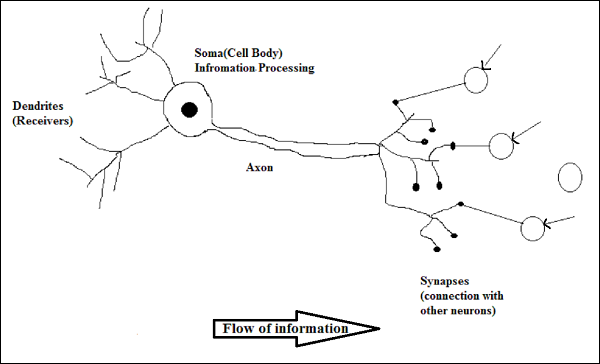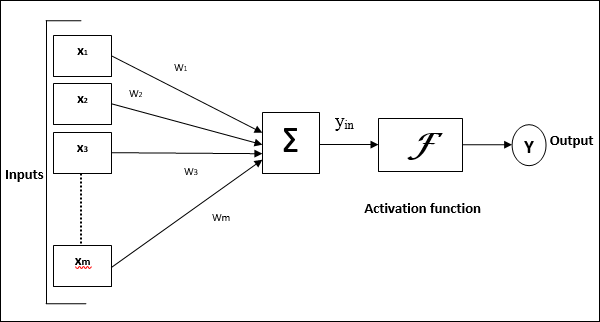# Artificial Neural Network - Basic Concepts

Neural networks are parallel computing devices, which is basically an attempt to make a computer model of the brain. The main objective is to develop a system to perform various computational tasks faster than the traditional systems. These tasks include pattern recognition and classification, approximation, optimization, and data clustering.

## What is Artificial Neural Network?

Artificial Neural Network (ANN) is an efficient computing system whose central theme is borrowed from the analogy of biological neural networks. ANNs are also named as “artificial neural systems,” or “parallel distributed processing systems,” or “connectionist systems.” ANN acquires a large collection of units that are interconnected in some pattern to allow communication between the units. These units, also referred to as nodes or neurons, are simple processors which operate in parallel.

Every neuron is connected with other neuron through a connection link. Each connection link is associated with a weight that has information about the input signal. This is the most useful information for neurons to solve a particular problem because the weight usually excites or inhibits the signal that is being communicated. Each neuron has an internal state, which is called an activation signal. Output signals, which are produced after combining the input signals and activation rule, may be sent to other units.

## A Brief History of ANN

The history of ANN can be divided into the following three eras −

### ANN during 1940s to 1960s

Some key developments of this era are as follows −

• 1943 − It has been assumed that the concept of neural network started with the work of physiologist, Warren McCulloch, and mathematician, Walter Pitts, when in 1943 they modeled a simple neural network using electrical circuits in order to describe how neurons in the brain might work.

• 1949 − Donald Hebb’s book, The Organization of Behavior, put forth the fact that repeated activation of one neuron by another increases its strength each time they are used.

• 1956 − An associative memory network was introduced by Taylor.

• 1958 − A learning method for McCulloch and Pitts neuron model named Perceptron was invented by Rosenblatt.

• 1960 − Bernard Widrow and Marcian Hoff developed models called "ADALINE" and “MADALINE.”

### ANN during 1960s to 1980s

Some key developments of this era are as follows −

• 1961 − Rosenblatt made an unsuccessful attempt but proposed the “backpropagation” scheme for multilayer networks.

• 1964 − Taylor constructed a winner-take-all circuit with inhibitions among output units.

• 1969 − Multilayer perceptron (MLP) was invented by Minsky and Papert.

• 1971 − Kohonen developed Associative memories.

• 1976 − Stephen Grossberg and Gail Carpenter developed Adaptive resonance theory.

### ANN from 1980s till Present

Some key developments of this era are as follows −

• 1982 − The major development was Hopfield’s Energy approach.

• 1985 − Boltzmann machine was developed by Ackley, Hinton, and Sejnowski.

• 1986 − Rumelhart, Hinton, and Williams introduced Generalised Delta Rule.

• 1988 − Kosko developed Binary Associative Memory (BAM) and also gave the concept of Fuzzy Logic in ANN.

The historical review shows that significant progress has been made in this field. Neural network based chips are emerging and applications to complex problems are being developed. Surely, today is a period of transition for neural network technology.

## Biological Neuron

A nerve cell (neuron) is a special biological cell that processes information. According to an estimation, there are huge number of neurons, approximately 1011 with numerous interconnections, approximately 1015.

### Schematic Diagram### Working of a Biological Neuron

As shown in the above diagram, a typical neuron consists of the following four parts with the help of which we can explain its working −

• Dendrites − They are tree-like branches, responsible for receiving the information from other neurons it is connected to. In other sense, we can say that they are like the ears of neuron.

• Soma − It is the cell body of the neuron and is responsible for processing of information, they have received from dendrites.

• Axon − It is just like a cable through which neurons send the information.

• Synapses − It is the connection between the axon and other neuron dendrites.

### ANN versus BNN

Before taking a look at the differences between Artificial Neural Network (ANN) and Biological Neural Network (BNN), let us take a look at the similarities based on the terminology between these two.

Biological Neural Network (BNN) Artificial Neural Network (ANN)
Soma Node
Dendrites Input
Synapse Weights or Interconnections
Axon Output

The following table shows the comparison between ANN and BNN based on some criteria mentioned.

Criteria BNN ANN
Processing Massively parallel, slow but superior than ANN Massively parallel, fast but inferior than BNN
Size 1011 neurons and 1015 interconnections 102 to 104 nodes (mainly depends on the type of application and network designer)
Learning They can tolerate ambiguity Very precise, structured and formatted data is required to tolerate ambiguity
Fault tolerance Performance degrades with even partial damage It is capable of robust performance, hence has the potential to be fault tolerant
Storage capacity Stores the information in the synapse Stores the information in continuous memory locations

## Model of Artificial Neural Network

The following diagram represents the general model of ANN followed by its processing.For the above general model of artificial neural network, the net input can be calculated as follows −

$$y_{in}\:=\:x_{1}.w_{1}\:+\:x_{2}.w_{2}\:+\:x_{3}.w_{3}\:\dotso\: x_{m}.w_{m}$$

i.e., Net input $y_{in}\:=\:\sum_i^m\:x_{i}.w_{i}$

The output can be calculated by applying the activation function over the net input.

$$Y\:=\:F(y_{in})$$

Output = function (net input calculated)